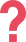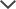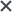## Sample Test Preview

### Verbal Reasoning Battery

The following example is a Verbal Analogy question. Verbal Analogy questions introduce a pair of words that are related in a certain way, along with a third word that is part of a different pair. You must choose a word from the answer choices that has the same relationship with the third word as the first two words have with each other.

Galaxy → Solar System: Sentence →

A. Letter
B. Word
C. Punctuation
D. Stars
E. CommaTestPrep-Online Developer's Tip:

In order to solve Verbal Analogy questions, you need to define the relationship between the first pair of words. The best way to do so is to form a sentence that describes the relationship.

Then, look for a word from the answer choices that has a similar relationship with the third word as the one you just defined. If the sentence that you formed accurately describes the relationship of the new pair, you have found a potential answer. If none of the answer choices fit, or if multiple answer choices relate to the third word in this way, you may have to adjust your sentence or think of a new one in order to include or exclude some of the potential answers.

A galaxy is made up of solar systems much like a sentence is made up of words

LetterPunctuation, and Comma are incorrect because they do not make up sentences. A sentence is not made up of many letters, but rather many words, and each word is made up of letters. The letters must first form words to make a sentence. Punctuation marks by themselves cannot form a sentence at all.

Stars is incorrect because it is only related to the first pair of words and not to the relevant third word.

The following example is a Verbal Classification question. Choose the word that best fits with the given group of words.

Halt    conclude    cease

A. Walk
B. Slowdown
C. Decelerate
D. Go
E. Pause

All the words above describe the action of stopping in a certain context.

The word ‘pause’ means to stop temporarily, so it fits the category.

Walk and go are incorrect because they describe things that move forward.

Slowdown and decelerate are incorrect because they describe something that is decreasing speed, but not actually stopping.

### Quantitative Reasoning Battery

The following example is a Number Series question. Number Series questions provide a series of numbers with a certain pattern or rule. You need to determine what the pattern is and use the rule to figure out which number will come next in the series.

12        21        29        38        46        55        63        72        ?

A. 79
B. 80
C. 81
D. 82
E. 83TestPrep-Online Developer's Tip:

To solve Number Series questions, you should start by thinking of a rule that describes the pattern in the set of numbers. For example, each number is greater than the previous number by 1.

Use the rule to determine which number will come next in the series. Then, look for the number you reached in the answer choices.

Sometimes patterns can be comprised of more than one rule. If a simple rule does not work, try out more complicated patterns. For example, the pattern may be to alternate between adding and subtracting specific numbers.

In this series, the pattern is as follows:

The pattern is: +9, +8, +9, +8, ...

The last two numbers in this series are 63 and 72. 72 is larger than 63 by 9 (+9), so the next number in the series should be larger than 72 by 8 (+8).

72 + 8 = 80

Therefore, 80 is the correct answer.

The following example is a Number analogy question. Choose the number that completes the third pair so that it demonstrates the same relationship as the first two pairs.

[15 → 50]        [10 → 35]        [7 → ?]

A. 21
B.
22
C.
25
D.
26
E.
32

For the first pair (15 → 50), the simplest possible way to turn in the first number (15) into the second number (50) is to add 35:

15 + 35 = 50

However, using the same formula of adding 35 will not work for the second pair in which 10 needs to become 35 (10 → 35):

10 + 35 = 45, not 35.

Therefore, we need to try a different formula for the first pair to find one that can work for all of them.

The next operation you should try in the first pair to turn 15 into 50 is multiplication (since subtraction and division would make 15 smaller, and 50 is bigger than 15).

However, since 15 can never become 50 no matter how many times you multiply it (15 → 30 → 45 → 60), this won't work either.

Now that we know that neither addition or multiplication work, the next step is to try a combination of both - multiplying 15 until it gets close to 50, then using addition to get the rest of the way there.

The closest you can get to 50 by multiplying 15 is 45 (15 x 3 = 45). Then to get to 50 from 45, you need to add 5.

15 x 3 + 5 = 50

Now you need to check if this formula of x 3 + 5 works for the next pair:

10 × 3 = 30, 30 + 5 = 35

Since this formula works for both the first and second pairs, we know that this is the formula that will get us the missing number in the third pair:

7 × 3 = 21, 21 + 5 = 26

Therefore, the correct answer is 26.

### Non-Verbal Reasoning Battery

The following example is a Figure Matrix question. Figure Matrix questions are either 2x2 or 3x3 matrices, comprised of different pictures. From the beginning to the end of each row and column, the pictures change in the same way or follow the same rule. You must choose an answer choice that goes in the empty box in the matrix that follows the same rule.TestPrep-Online Developer's Tip:

To solve Figure Matrices questions, start by examining how the series of shapes change across the rows and down the columns within the matrix. The direction in which you examine the question depends on where you can most easily visualize the relationship.

Test the relationship rule on each answer choice and eliminate answers that do not fit. If more than one answer choice fits the rule, look for a more accurate rule. Remember that sometimes there can be more than one applicable rule.

In this matrix:
Across a row (from left to right), first one shape is removed from the upper-right corner, then two shapes are removed from the top. The remaining shapes stay in the same position.
Down a column, the shape changes, and an extra shape is added to each successive frame.

We can eliminate answer choices B, C, and D because they contain the wrong number of shapes. We can also eliminate answer choice E because the top two shapes should be removed, not the bottom two.

Therefore, answer choice A is the only possible correct solution.

The following example is a Figure Classification question. Choose the answer which shares the same connection/characteristic as the three given images.All the arrows have black borders. Therefore, the correct answer will be the arrow with a black border.

Choices 1 and 5 have blue borders. Choice 2 has an orange border. Choice 3 has a red border.

Hence, 4 is the only choice that matches the pattern and it is the correct answer.

### Spatial Ability Reasoning Battery

The following example is a Figure Analysis question. Figure Analysis questions show a paper folded several times and then punched with holes. The answer choices contain unfolded papers with punched-in holes. You need to determine which of the answer choices is the final product of the unfolded punched-in paper.TestPrep-Online Developer's Tip:

To solve Paper Folding questions, start by eliminating answer choices that do not contain the original holes shown in the question.

Next, determine the number of holes that should be on the unfolded paper. For example, if the paper was folded once into two layers, each hole will go through both layers so there should be twice the number of holes. Remember that each folding of the paper doubles the number of layers that are being folded (and does not just add one layer as in the first folding). However, pay attention to which part of the paper is being folded. If the first fold does not cover the whole paper, and the second fold does not overlap with the first fold, there is no reason to multiply the layers twice.

Folding of the paper creates a symmetry line, meaning that punching holes in a folded paper make a mirror image along that line (after unfolding). Therefore, try to imagine how the holes will be reflected on the other side of the paper each time it is unfolded. Each hole will be the same distance away from the crease as its counterpart, but in the opposite direction, in a straight line that crosses the line of symmetry at a right angle.

When confused, you can always draw the problem on a square sheet of paper.

• The paper was folded from the two left corners towards the centre of the square.
• Then, four holes were punched
• Two of the holes go through two layers of paper and the other two holes go through the unfolded single layer, so the answer should include six holes: (2 holes x 2 layers) + (2 holes x 1 layer) = 6 holes.

Answer E contains all four original holes, as well as two additional holes in the mirror image across the crease to the holes that were punched through two layers of paper, so it is the correct answer.

Answers A and B are incorrect because they either have more or less than six holes.

Answer C is incorrect because the two central holes are placed on the right half of the square, unlike the original left side holes.

Answer D is incorrect because the holes on the left side of the square are too close to each other.

The following example is a Figure Classification question. Choose the answer choice that contains the given shape. The shape must keep its size and orientation.TestPrep-Online offers an accurate CAT4 Level D Practice Pack, featuring a comprehensive study guide and hundreds of practice questions, as well as thorough explanations and solving tips.

## Get Test-Taking Tips for Your Child's Upcoming Exam

The CAT4 and other trademarks are the property of their respective trademark holders. None of the trademark holders are affiliated with TestPrep-Online or this website.

#### Free CAT4 Level D Test

Reviews

Need HelpNeed Help